## Estimate Constrained Values of a Lookup Table

### Objectives

This example shows how to estimate constrained values of a lookup table in the Parameter Estimation tool. Apply monotonically increasing constraints to the lookup table output values, and use the Parameter Estimation tool to estimate the table values.

In this example, use `lookup_increasing.mat`, which contains the measured I/O data for estimating the lookup table values. The MAT-file includes the following variables:

• `xdata1` — Input data consisting of 602 uniformly sampled data points in the range `[-5,5]`.

• `ydata1` — Output data corresponding to the input data samples.

• `time1` — Time vector.

Use the I/O data to estimate monotonically increasing output values of the lookup table in the `lookup_increasing` Simulink® model.

### Lookup Table Output

1. Open the lookup table model by typing the following command at the MATLAB® prompt:

`lookup_increasing`

This command opens the Simulink model, and loads the estimation data in the MATLAB workspace.2. View the table output values by double-clicking the Lookup Table block.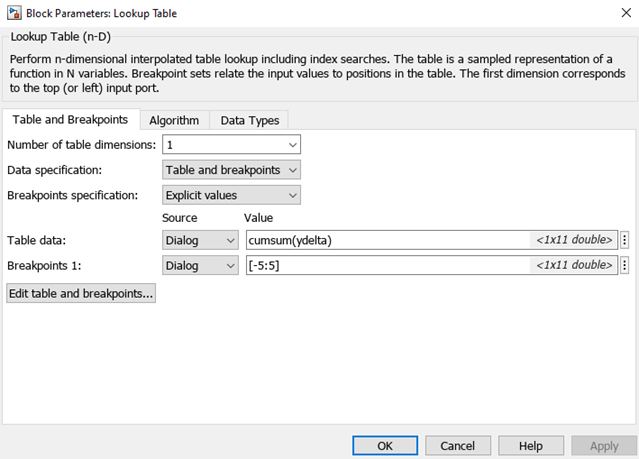The table contains 11 output values at breakpoints `[-5:5]`, specified in the Function Block Parameters dialog box. To learn more about how to specify the table values, see Enter Breakpoints and Table Data (Simulink).

The Table data field shows that the table output values are the cumulative sum of the values stored in variable `ydelta`. Thus, if yn are the 11 table output values, `ydelta` is (y1, y2y1, y3y2, ..., y11`y10`). The initial `ydelta` values are loaded from `lookup_increasing.mat`.

The initial table output values are not monotonically increasing. To ensure monotonically increasing table output values, the difference between adjacent table output values should be positive. To do so, estimate `ydelta` in the Parameter Estimation tool using the measured I/O estimation data, and constrain `ydelta(2:end)` to be positive during estimation.

### Estimate the Monotonically Increasing Table Values Using Default Settings

1. Open a parameter estimation session.

In the Simulink model, select Parameter Estimation from the Apps tab, in the gallery, under Control Systems to open a session with the name lookup_increasing in the Parameter Estimation tool.2. Create an experiment and import the I/O data.

On the Parameter Estimation tab, click . Type `[time1,ydata1]` in Outputs and `[time1,xdata1]` in Inputs of the Edit Experiment dialog box. Click . A new experiment with name `Exp` is created in the Experiments area of the tool. Rename the experiment `EstimationData` by right-clicking the default experiment name, `Exp`, and selecting `Rename`. For more information, see Import Data for Parameter Estimation.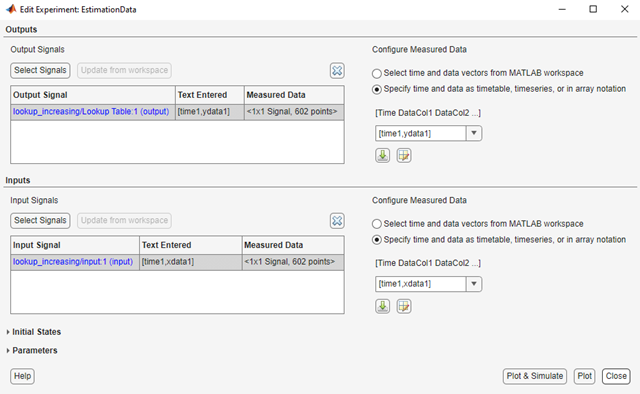3. Run an initial simulation to view the measured data, simulated model values, and the initial table values by typing the following commands at the MATLAB prompt.

```sim('lookup_increasing') figure(1); plot(xdata1,ydata1,'m*',xout,yout,'b^') hold on; plot(-5:5,cumsum(ydelta),'k','LineWidth',2) xlabel('Input Data'); ylabel('Output Data'); legend('Measured data','Initial simulated data','Initial table output')```The initial table output values and simulated data do not match the measured data.

4. Select parameter for estimation.

On the Parameter Estimation tab, click . The Edit: Estimated Parameters dialog box opens. In the Parameters Tuned for all Experiments panel, click to open the Select Model Variables dialog box. Check the box next to `ydelta`, and click .The `ydelta` values are selected for estimation by default in the Edit: Estimated Parameters dialog box.5. Apply a monotonically increasing constraint on the table output values. For more details about the table, see Lookup Table Output.

In the Edit: Estimated Parameters dialog box, click the arrow next to the `ydelta` values. In the expanded menu, set Minimum `ydelta` values to `[-Inf,zeros(1,10)]`. Thus, while the first value in `ydelta` can by anything, subsequent values which are the difference between adjacent table output values, must be positive.6. Select `EstimationData` experiment for estimation.

On the Parameter Estimation tab, click . By default, `EstimationData` is selected for estimation. If not, check the box under the Estimation column, and click .7. Estimate the table values using default settings.

On the Parameter Estimation tab, click .

The Parameter Trajectory plot shows the change in the parameter values at each iteration.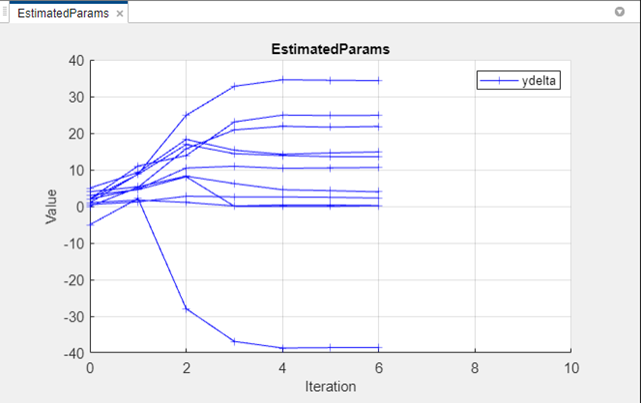The Estimation Progress Report shows the iteration number, number of times the objective function is evaluated, and value of the cost function at the end of each iteration.The estimated parameters are saved in a new variable, `EstimatedParams`, in the Results area of the tool. To view the estimated parameters, right-click `EstimatedParams` and select Open.The estimated `ydelta(2:end)` values are positive. Thus, the output of the table, which is the cumulative sum of the values stored in `ydelta`, is monotonically increasing.

### Validate the Estimation Results

After you estimate the table values, as described in Estimate the Monotonically Increasing Table Values Using Default Settings, you use another measured data set to validate and check that you have not over-fit the model. You can plot and examine the following plots to validate the estimation results:

• Residuals plot

• Measured and simulated data plots

1. Create an experiment to use for validation and import the validation I/O data.

On the Parameter Estimation tab, click . Type `[time2,ydata2]` in Outputs and `[time2,xdata2]` in Inputs of the Edit Experiment dialog box. Name the experiment `ValidationData` by right-clicking the default experiment name, `Exp`, in the Experiments area of the tool, and selecting `Rename`. For more information, see Import Data for Parameter Estimation.

2. Select the experiment for validation.

Click on the tab. The `ValidationData` experiment is selected for estimation by default. Clear Estimation and select the box for Validation.3. Select the results to validate.

On the Validation tab, click . Clear `Use current parameter values`, select `EstimatedParams`, and click .4. Select the plots to display during validation.

The Parameter Estimation tool displays the experiment plot after validation by default. Add the residuals plot by selecting the corresponding box on the Validation tab.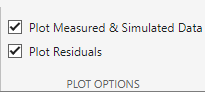Click .

5. Examine the plots.

1. The experiment plot shows the data simulated using estimated parameters agrees with the measured validation data.2. To view the residuals plot, click Residual plot: ValidationData tab.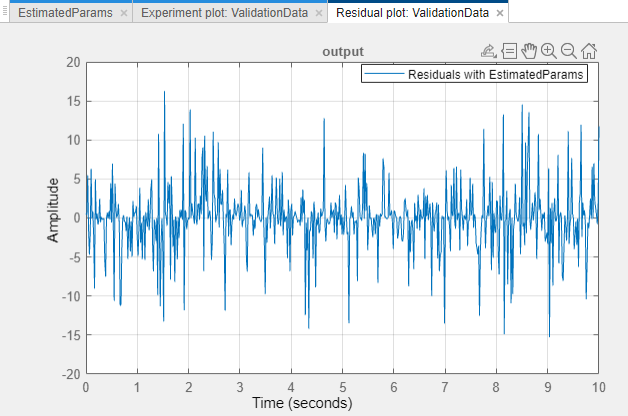The residuals, which show the difference between the simulated and measured data, lie within 15% of the maximum output variation. This indicates a good match between the measured and simulated table data values.

3. Plot and examine the validation data, simulated data, and estimated table values.

```sim('lookup_increasing') figure(2); plot(xdata2,ydata2,'m*',xout,yout,'b^') hold on; plot(-5:5,cumsum(ydelta),'k','LineWidth', 2) xlabel('Input Data'); ylabel('Output Data'); legend('Validation data','Simulated data','Table output values');```The table output values match both the measured data and the simulated table values. The table output values cover the entire range of input values, which indicates that all the lookup table values have been estimated.

## Related Topics